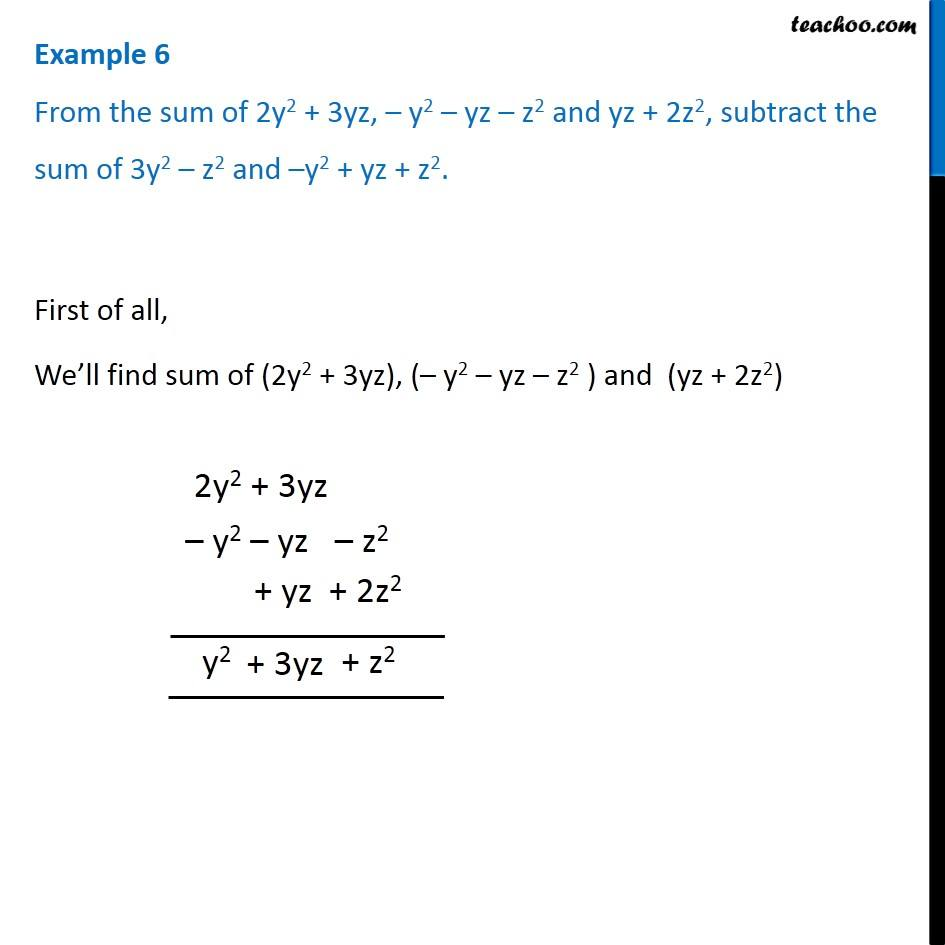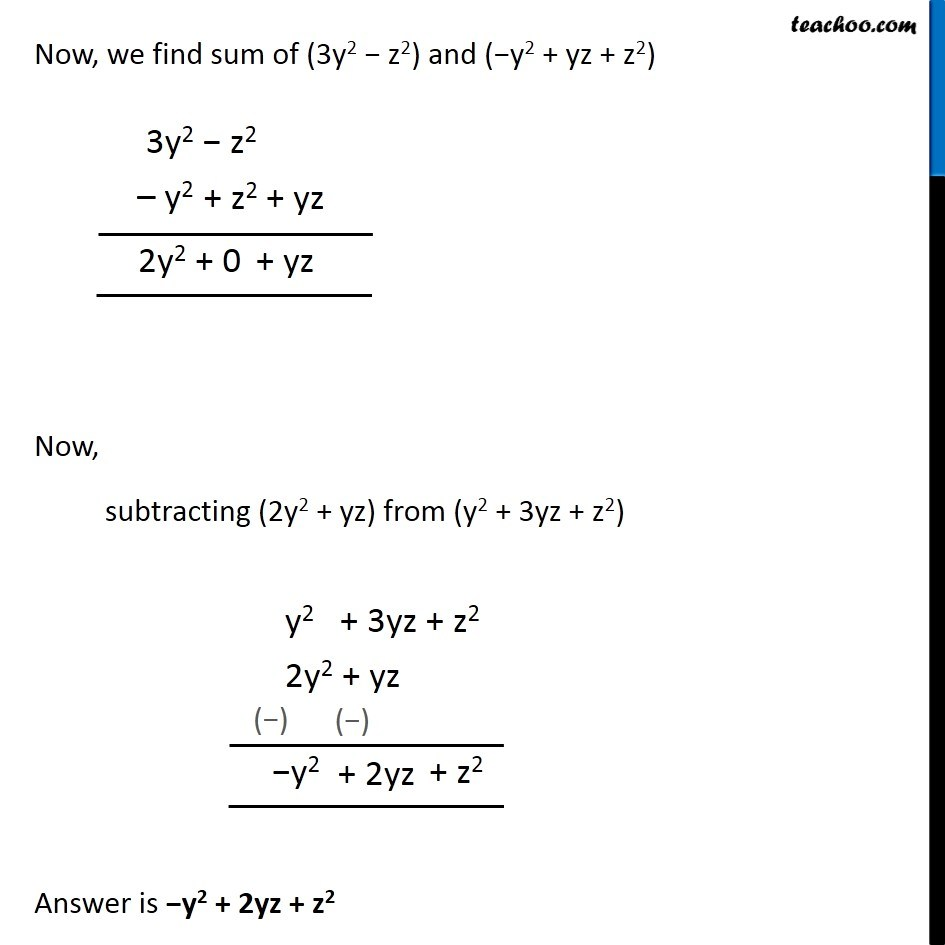Examples

Chapter 10 Class 7 Algebraic Expressions
Serial order wiseLearn in your speed, with individual attention - Teachoo Maths 1-on-1 Class

### Transcript

Question 3 From the sum of 2y2 + 3yz, – y2 – yz – z2 and yz + 2z2, subtract the sum of 3y2 – z2 and –y2 + yz + z2. First of all, We’ll find sum of (2y2 + 3yz), (– y2 – yz – z2 ) and (yz + 2z2)